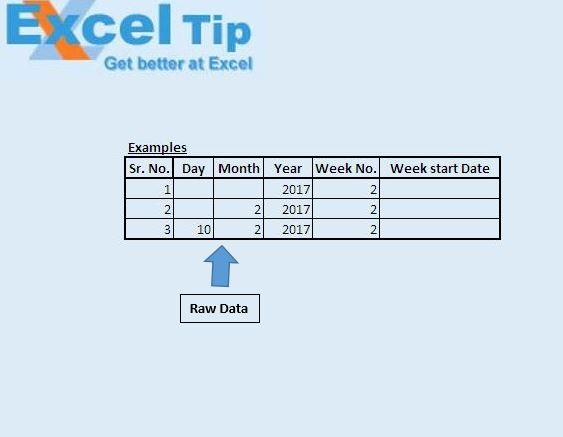# Calculate a week start date using VBA in Microsoft Excel

In this article, we will create a user defined function (UDF) or custom function to find the first date for any given week, considering Monday as the first day of the week. We will create a custom function which will return the first date of the week after specified number of week from the given year, month or date.

In this article, raw data consists of week number, day, month and year. Custom function will have day, month, year and week number as input parameters. Based on these input values, function will return the first day for the week.Syntax for custom function “WeekStartDate” is

WeekStartDate(WeekNumber, Year, Month, Day)

Month and Day are optional parameters.

This function assumes Monday as first day of the week.

WeekStartDate function returns the first day of the week after specified number of weeks from the date specified by the Day, Month and Year parameter. If Day parameter is not specified then it will return the first day of the week after specified number of week in the month specified by the Month parameter of the specified year. If both Month and Day parameter are not specified then it will return the first day of specified week of the year.Logic explanation

We have created “WeekStartDate” custom function to find the first day of the week. Logic used for this function is that if week day of the given date is less than 4, then current week will be considered as the first week. Otherwise, if week day of the given date is greater than 4 then next week will be considered as first week.

Code explanation

DateSerial(intYear, intMonth, intDay)

DateSerial function is used to get date from the day, month and year value.

Weekday(FromDate, vbMonday)

Weekday function is used to get the week day for the date, considering Monday as the first day of the week.

If WKDay > 4 Then

WDays = (7 * intWeek) - WKDay + 1

Else

WDays = (7 * (intWeek - 1)) - WKDay + 1

End If

Above code is used to find the number of days to be included to find the first date of the week. If week day value is less than 4, then current week is considered as first week. That is why we have subtracted 1 i.e. (intWeek - 1). 1 is added as we want to find the first day of the week.

```
Option Explicit

Function WeekStartDate(intWeek As Integer, intYear As Integer, Optional intMonth As Integer = 1, Optional intDay As Integer = 1)

'Declaring variables
Dim FromDate As Date, lngAdd As Long
Dim WKDay, WDays As Integer

WDays = 0

'Checking that year should not have negative value
If intYear < 1 Then
WeekStartDate = "Year cann't have negative value"
Exit Function
End If

'Calculating the date
FromDate = DateSerial(intYear, intMonth, intDay)

'Getting the week day of the specified date considering monday as first day
WKDay = Weekday(FromDate, vbMonday)

'If value of week day is less than 4 then subtracting 1 from the week number
If WKDay > 4 Then
WDays = (7 * intWeek) - WKDay + 1
Else
WDays = (7 * (intWeek - 1)) - WKDay + 1
End If

'Return the first day of the week
WeekStartDate = FromDate + WDays

End Function

```